# Math Models of Hierarchy: Dominance, Dynamics, and Data

Smith College | April 6th, 2023

Dr. Phil Chodrow
Department of Computer Science
Middlebury College

#### Hi! I’m Phil.

I am an
applied mathematician
and
STEM educator.

I like…

• Mathematics of complex systems
• Network science
• Ethics and technology
• Tea
• Star Trek: Deep Space 9
• Effective pedagogy

I’m a new professor in the department of computer science at Middlebury College in Middlebury, Vermont.

I teach machine learning, discrete math, introductory computing, and network science.

### This is a talk about hierarchy

the classification of a group of people according to ability or to economic, social, or professional standing

#### Examples of hierarchies

Standings in competitions

Rankings of colleges, PhD programs, etc.

Popularity

Dominance in animal societies

#### 3. What makes science joyful (for me)?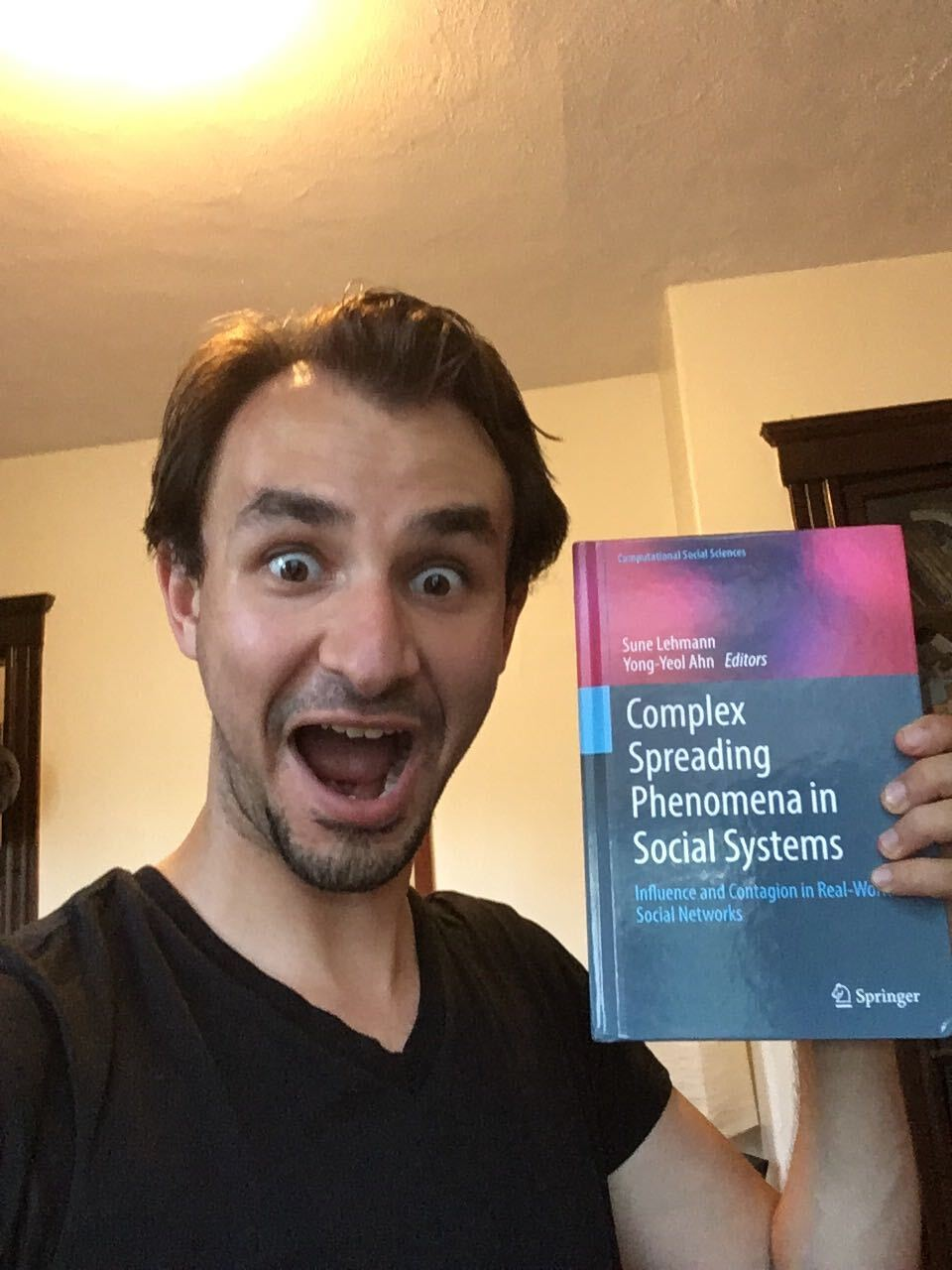#### Collaboration Origin Story

Complex Networks Winter Workshop 2019

### 18 Months Later…

Mari Kawakatsu
UPenn and Princeton

Nicole Eikmeier
Grinnell

Dan Larremore
CU Boulder

#### A model of prestige-based hierarchy

We have $n$ agents.

These agents can “endorse” each other.

When agent $i$ endorses agent $j$, we interpret this as agent $i$ signalling that agent $j$ is of high prestige.

“Endorsements:”

• Player $i$ loses a match to player $j$.
• University $i$ hires a scholar trained at university $j$.
• Individual $i$ claims that $j$ is their friend.

#### A model of prestige-based hierarchy

A state of the model is a matrix $\mathbf{A}^{(t)} \in \mathbb{R}^{n\times n}$ of endorsements.

$a_{ij}^{(t)}$ is the weighted number times that $i$ has endorsed $j$.

At each timestep, we update $\mathbf{A}^{(t)}$: $\mathbf{A}^{(t+1)} = \lambda \mathbf{A}^{(t)} + (1-\lambda) \Delta^{(t)}$ where $\Delta^{(t)}$ is the matrix of new endorsements.

$\lambda$ acts as the rate of exponentially decaying system memory.

#### New Endorsements

Agents endorse other agents that they perceive as being of high prestige.

Prestige is measured by a score function $\sigma: \mathbf{A}^{(t)} \mapsto \mathbf{r}^{(t)} \in \mathbb{R}^n$.

Each agent $i$ computes a utility of endorsing agent $j$ in terms of the score vector $\mathbf{r}^{(t)}$. Our utility is: $u_{ij}(\mathbf{r}) = \beta_1 s_j + \beta_2 (r_i - r_j)^2.$

Agent $i$ endorses $j$ at time $t+1$ with probability $p_{ij}^{(t)} = \frac{e^{u_{ij}(\mathbf{r}^{(t)})}}{\sum_j e^{u_{ij}(\mathbf{r}^{(t)})}}$

All new endorsements get collected into update matrix $\Delta^{(t)}$. Then we do our main update: $\mathbf{A}^{(t+1)} = \lambda \mathbf{A}^{(t)} + (1-\lambda) \Delta^{(t)}\;.$

We allow $m\in \mathbb{Z}$ endorsements per round.

#### New Endorsements

Agents endorse other agents that they perceive as being of high prestige.

Prestige is measured by a score function $\sigma: \mathbf{A}^{(t)} \mapsto \mathbf{r}^{(t)} \in \mathbb{R}^n$.

Each agent $i$ computes a utility of endorsing agent $j$ in terms of the score vector $\mathbf{r}^{(t)}$. Our utility is: $u_{ij}(\mathbf{r}) = \color{#FFD447}{\beta_1} s_j + \color{#59C9A5}{\beta_2} (r_i - r_j)^2.$

Agent $i$ endorses $j$ at time $t+1$ with probability $p_{ij}^{(t)} = \frac{e^{u_{ij}(\mathbf{r}^{(t)})}}{\sum_j e^{u_{ij}(\mathbf{r}^{(t)})}}$

We allow $m\in \mathbb{Z}$ endorsements per round.

$\beta_1$ encodes a preference to endorse high-prestige agents. Higher value: more likely for endorsements to concentrate at the top.

$\beta_2$ encodes a preference to endorse agents that are similar in prestige to you. Negative value: more likely for endorsements to “travel” only a little way up the hierarchy.

The score function $\sigma$ maps the matrix of endorsements $\mathbf{A} \in \mathbb{R}^{n\times n}$ to a vector of scores $\mathbf{s} \in \mathbb{R}^n$. There are lots of choices!

##### In-Degree

$s_i$ is the number of times that agent $i$ h as been endorsed: $\mathbf{r} = \mathbb{1}^T\mathbf{A}$

##### PageRank

$\mathbf{r}$ is the eigenvector with eigenvalue $1$ of the matrix $\mathbf{P}$ with entries $p_{ij} = (1-\alpha)\frac{a_{ij}}{d_i} + \frac{\alpha}{n}$

##### SpringRank

$\mathbf{r}$ is the unique solution of the system $\left[\mathbf{L} - \alpha \mathbf{I}\right]\mathbf{r} = \mathbf{D}^{\mathrm{in}} - \mathbf{D}^{\mathrm{out}}$

$\mathbf{D}^{\mathrm{in}} = \mathrm{diag}(\mathbb{1}^T\mathbf{A})$ and $\mathbf{D}^{\mathrm{out}} = \mathrm{diag}(\mathbf{A}\mathbb{1})$

#### 1. Can hierarchies sustain themselves on the basis of nothing but prestige?

Yes, and there is a phase transition (qualitative behavior shift) depending on the parameters.

#### Model do?

$\beta_1$ preference for prestige.

• $\beta_1$ small: fluctuating egalitarianism.
• $\beta_1$ large: emergence of time-varying hierarchies.

$\beta_2$ preference for proximity.

• $\beta_2 < 0$: stabilization of hierarchical ranks.

#### Two math problems

Dynamics: can we mathematically characterize when we observe egalitarianism and when we observe hierarchy in terms of the score function, $\beta_1$, and $\beta_2$?

Data science: can we determine which score functions and parameters best describe the behavior of real systems?

#### Theorem: KCEL ’21

Consider the deterministic function $\mathbf{f}(\mathbf{r}, \mathbf{A}) = \lim_{\lambda \rightarrow 1} \frac{\mathbb{E}[\mathbf{r}|\mathbf{A}] - \mathbf{r}}{1 - \lambda}\;.$

This function has an egalitarian fixed point, where all ranks are the same. This point fixed point is linearly stable iff $\beta_1 < \beta_1^c$:

$\beta_1^c = \begin{cases} 2\sqrt{\frac{n}{m}} &\quad \text{Root-Degree} \\ 1/\alpha_p &\quad \text{PageRank} \\ 2 + \alpha_s\frac{n}{m} &\quad \text{SpringRank}. \end{cases}$

#### Theorem: KCEL ’21

Consider the deterministic function $\mathbf{f}(\mathbf{r}, \mathbf{A}) = \lim_{\lambda \rightarrow 1} \frac{\mathbb{E}[\mathbf{r}|\mathbf{A}] - \mathbf{r}}{1 - \lambda}\;.$

This function has an egalitarian fixed point, where all ranks are the same. This point fixed point is linearly stable iff $\beta_1 < \beta_1^c$:

$\beta_1^c = \begin{cases} 2\sqrt{\frac{n}{m}} &\quad \text{Root-Degree} \\ 1/\alpha_p &\quad \text{PageRank} \\ 2 + \alpha_s\frac{n}{m} &\quad \text{SpringRank}. \end{cases}$

#### Linear Stability

Heuristically, a fixed point $\mathbf{x}_0$ of some dynamics is stable if, when you perturb the the system to some nearby point, it eventually comes back to $\mathbf{x}_0$.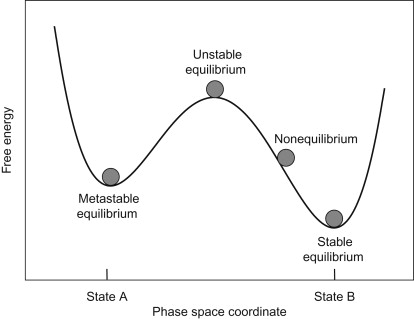Image credit: Desmond Winterborne

#### Theorem: KCEL ’21

Consider the deterministic function $\mathbf{f}(\mathbf{r}, \mathbf{A}) = \lim_{\lambda \rightarrow 1} \frac{\mathbb{E}[\mathbf{r}|\mathbf{A}] - \mathbf{r}}{1 - \lambda}\;.$

This function has an egalitarian fixed point, where all ranks are the same. This point fixed point is linearly stable iff $\beta_1 < \beta_1^c$:

$\beta_1^c = \begin{cases} 2\sqrt{\frac{n}{m}} &\quad \text{Root-Degree} \\ 1/\alpha_p &\quad \text{PageRank} \\ 2 + \alpha_s\frac{n}{m} &\quad \text{SpringRank}. \end{cases}$

#### Linear Stability

Here, stability of the egalitarian fixed point of $\mathbf{f}$ is governed by the Jacobian matrix of partial derivatives:

$\mathbf{J} = \left[\begin{matrix}\frac{\partial f_1}{\partial r_1} & \frac{\partial f_1}{\partial r_2} & \cdots & \frac{\partial f_1}{\partial r_n} \\ \frac{\partial f_2}{\partial r_1} & \frac{\partial f_2}{\partial r_2} & \cdots & \frac{\partial f_2}{\partial r_n} \\ \vdots & \vdots & \ddots & \vdots \\ \frac{\partial f_n}{\partial r_1} & \frac{\partial f_n}{\partial r_2} & \cdots & \frac{\partial f_n}{\partial r_n} \end{matrix}\right]$

Fixed point is linearly stable if $\mathbf{J}$ only has eigenvalues with negative real part.

So, we can assess the stability of egalitarianism in this system with calculus + linear algebra.

#### Theorem: KCEL ’21

Consider the deterministic function $\mathbf{f}(\mathbf{r}, \mathbf{A}) = \lim_{\lambda \rightarrow 1} \frac{\mathbb{E}[\mathbf{r}|\mathbf{A}] - \mathbf{r}}{1 - \lambda}\;.$

This function has an egalitarian fixed point, where all ranks are the same. This point fixed point is linearly stable iff $\beta_1 < \beta_1^c$:

$\beta_1^c = \begin{cases} 2\sqrt{\frac{n}{m}} &\quad \text{Root-Degree} \\ 1/\alpha_p &\quad \text{PageRank} \\ 2 + \alpha_s\frac{n}{m} &\quad \text{SpringRank}. \end{cases}$

#### Theorem: KCEL ’21

Consider the deterministic function $\mathbf{f}(\mathbf{r}, \mathbf{A}) = \lim_{\lambda \rightarrow 1} \frac{\mathbb{E}[\mathbf{r}|\mathbf{A}] - \mathbf{r}}{1 - \lambda}\;.$

This function has an egalitarian fixed point, where all ranks are the same. This point fixed point is linearly stable iff $\beta_1 < \beta_1^c$:

$\beta_1^c = \begin{cases} 2\sqrt{\frac{n}{m}} &\quad \text{Root-Degree} \\ 1/\alpha_p &\quad \text{PageRank} \\ 2 + \alpha_s\frac{n}{m} &\quad \text{SpringRank}. \end{cases}$

#### Theorem: KCEL ’21

Consider the deterministic function $\mathbf{f}(\mathbf{r}, \mathbf{A}) = \lim_{\lambda \rightarrow 1} \frac{\mathbb{E}[\mathbf{r}|\mathbf{A}] - \mathbf{r}}{1 - \lambda}\;.$

This function has an egalitarian fixed point, where all ranks are the same. This point fixed point is linearly stable iff $\beta_1 < \beta_1^c$:

$\beta_1^c = \begin{cases} 2\sqrt{\frac{n}{m}} &\quad \text{Root-Degree} \\ 1/\alpha_p &\quad \text{PageRank} \\ 2 + \alpha_s\frac{n}{m} &\quad \text{SpringRank}. \end{cases}$

#### Learnings

1. The stability of egalitarianism depends on the prestige preference $\beta_1$ but not on the proximity preference $\beta_2$.
2. The stability of egalitarianism also depends on the score function used.
3. In some score functions, in some parameter regimes, egalitarianism and hierarchy are both possible (bistability).

#### 2. Can we infer how agents interact with hierarchies from data?

Yes: we can find most likely parameters and score functions.

Because of the specific form of the probability $p_{ij}^{(t)}$, this boils down to logistic regression.

Recall that we had this funny looking function that defined a probability of agent $i$ endorsing agent $j$:

$p_{ij}^{(t)} = \frac{e^{u_{ij}(\mathbf{r}^{(t)})}}{\sum_j e^{u_{ij}(\mathbf{r}^{(t)})}}$

This lets us assign a likelihood to the observed data as a function of the parameters:

$\mathcal{L}(\lambda, \beta) = \sum_{t = 0}\sum_{i, j \in \mathcal{N}}a_{ij}^{(t)} \log p_{ij}^{(t)}$

Method of maximum-likelihood: find $\lambda$ and $\beta$ to make this function large.

### Data:

Math PhD Exchange¹: University A “endorses” B by hiring a PhD trained at B, over 50 years.
Monk Parakeets²: Parakeet A “endorses” B by losing a fight to B, over 4 observation periods.
Newcomb Fraternity³: Fraternity brother A “endorses” B by stating that they like B on a survey, over a semester (15 weeks).

¹D. Taylor, S. A. Meyers, A. Clauset, M. A. Porter, P. J. Mucha, Eigenvector-based centrality measures for temporal networks. Multiscale Model. Simul. 15, 537–574 (2017).
North Dakota State University Department of Mathematics, Data from “The Mathematics Genealogy Project.” (link)
²E. A. Hobson, S. DeDeo, Social feedback and the emergence of rank in animal society. PLoS Comput. Biol. 11, e1004411 (2015)
³T. Newcomb, The Acquaintance Process (Holt, Reinhard, and Winston, New York, NY, 1961).

Recall that we had this funny looking function that defined a probability of agent $i$ endorsing agent $j$:

$p_{ij}^{(t)} = \frac{e^{u_{ij}(\mathbf{r}^{(t)})}}{\sum_j e^{u_{ij}(\mathbf{r}^{(t)})}}$

This lets us assign a likelihood to the observed data as a function of the parameters:

$\mathcal{L}(\lambda, \beta) = \sum_{t = 0}\sum_{i, j \in \mathcal{N}}a_{ij}^{(t)} \log p_{ij}^{(t)}$

Method of maximum-likelihood: find $\lambda$ and $\beta$ to make this function large.

### Data:

Math PhD Exchange¹: University A “endorses” B by hiring a PhD trained at B, over 50 years.
Monk Parakeets²: Parakeet A “endorses” B by losing a fight to B, over 4 observation periods.
Newcomb Fraternity³: Fraternity brother A “endorses” B by stating that they like B on a survey, over a semester (15 weeks).

¹D. Taylor, S. A. Meyers, A. Clauset, M. A. Porter, P. J. Mucha, Eigenvector-based centrality measures for temporal networks. Multiscale Model. Simul. 15, 537–574 (2017).
North Dakota State University Department of Mathematics, Data from “The Mathematics Genealogy Project.” (link)
²E. A. Hobson, S. DeDeo, Social feedback and the emergence of rank in animal society. PLoS Comput. Biol. 11, e1004411 (2015)
³T. Newcomb, The Acquaintance Process (Holt, Reinhard, and Winston, New York, NY, 1961).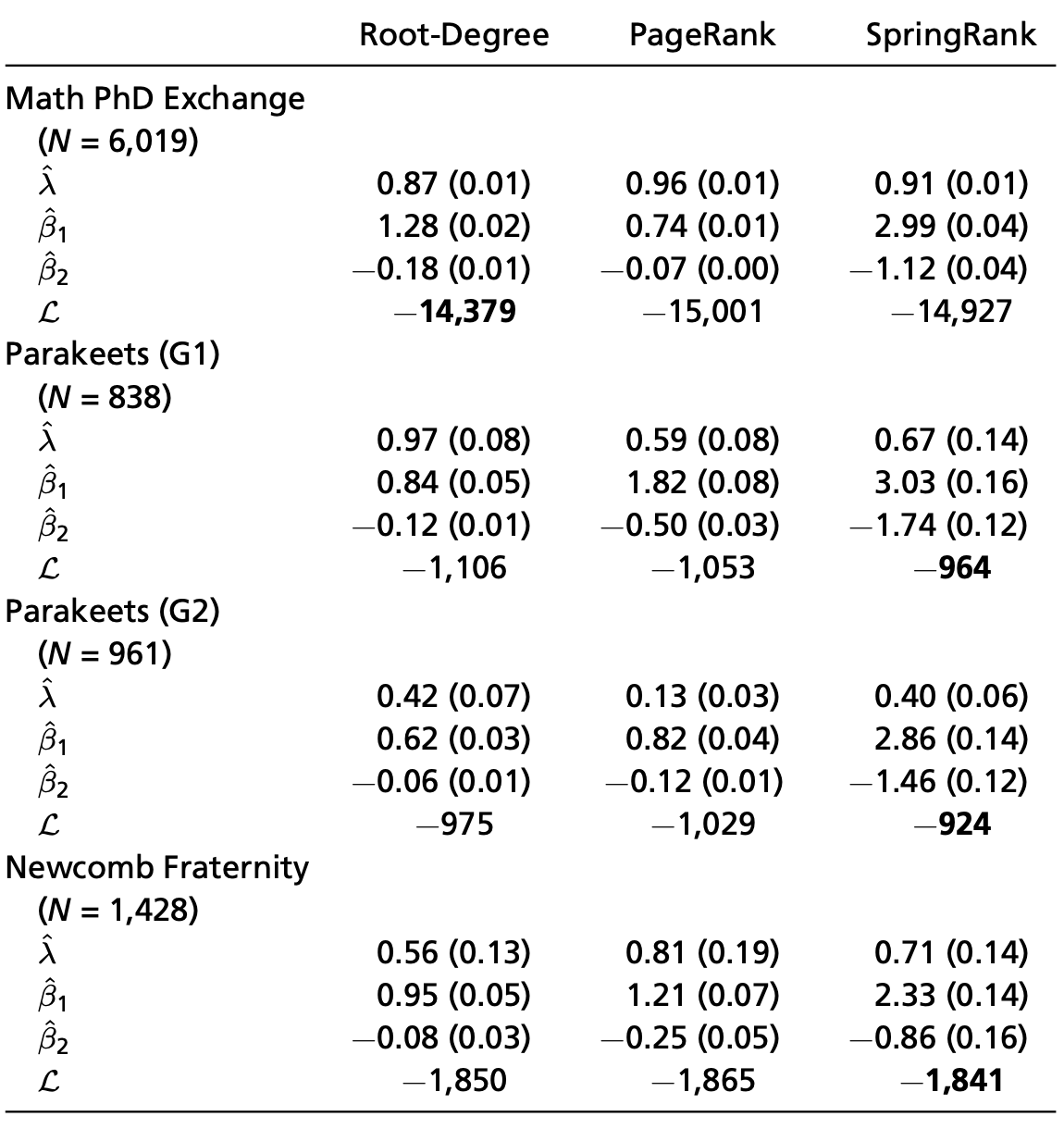#### Are we in the hierarchical regime?

Theorem from before: Egalitarianism is stable iff $\beta_1 < \beta_1^c$, where

$\beta_1^c = \begin{cases} 2\sqrt{\frac{n}{m}} &\quad \text{Root-Degree} \\ 1/\alpha_p &\quad \text{PageRank} \\ 2 + \alpha_s\frac{n}{m} &\quad \text{SpringRank}. \end{cases}$

Math PhD exchange: bistable $-$ both egalitarianism and hierarchy are possible.

Parakeets + Newcomb Frat: hierarchical.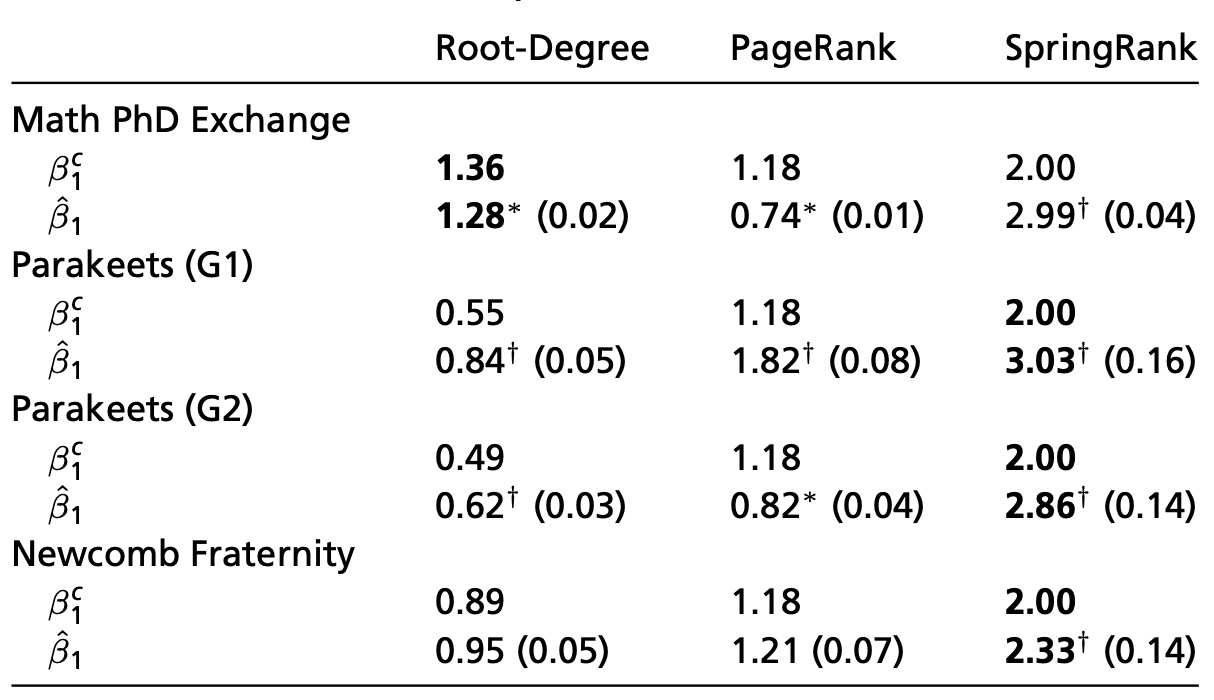##### It matters how we compute the ranks!

Placement share, Root-Degree, and PageRank: MIT dominates, esp. recently.

SpringRank: Favors Harvard, Stanford, Princeton, and Berkeley (based on prestigious placements of their graduates).

Different estimates of fluidity in ranks.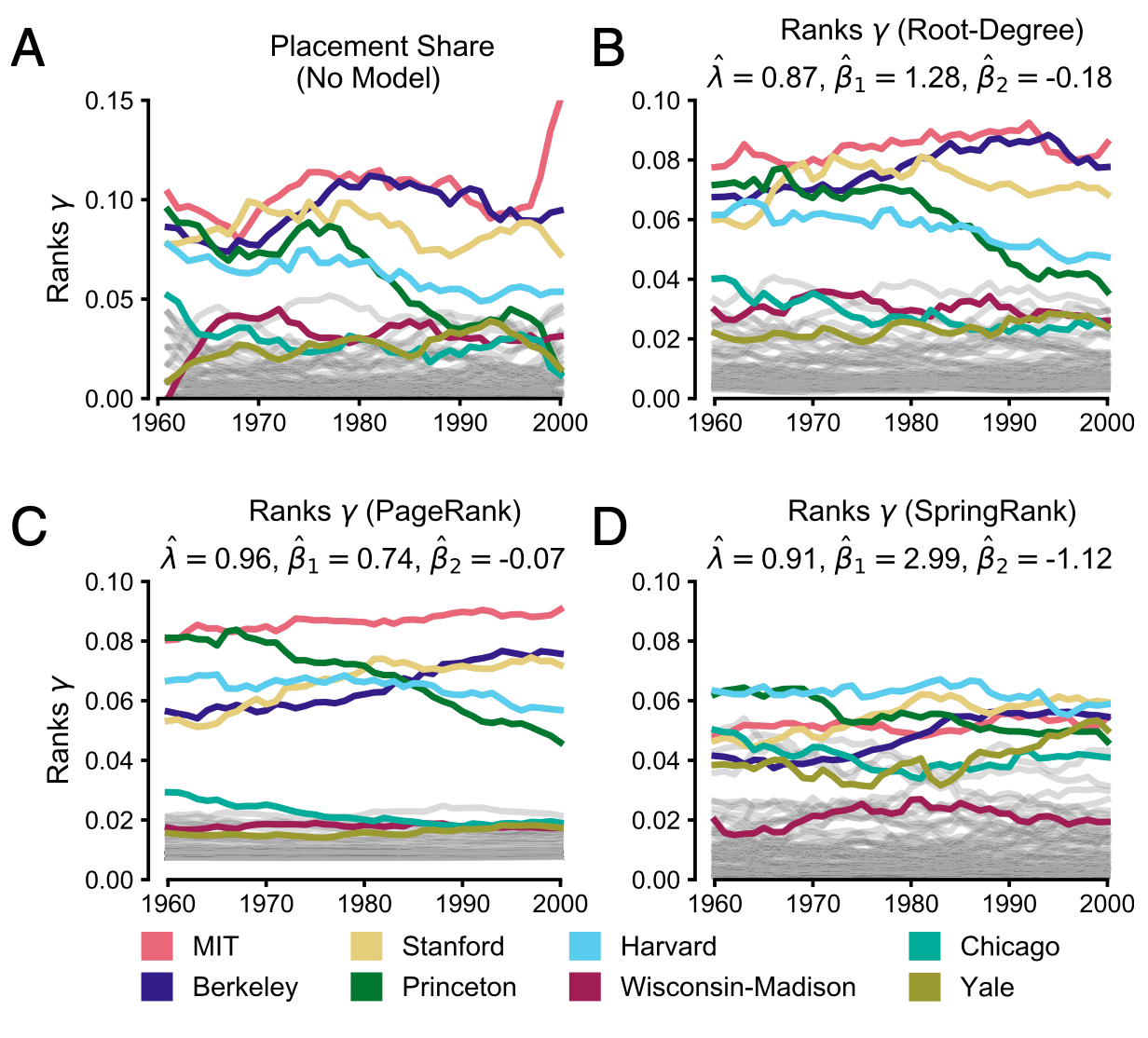### Ranks ⟳ Decisions

We wrote a simple math model of hierarchies emerging from feedback loops.

Feedback loops can generate stable hierarchies, even when are no meaningful differences between agents.

Some systems are near criticality: small interventions could help to promote equality and equity.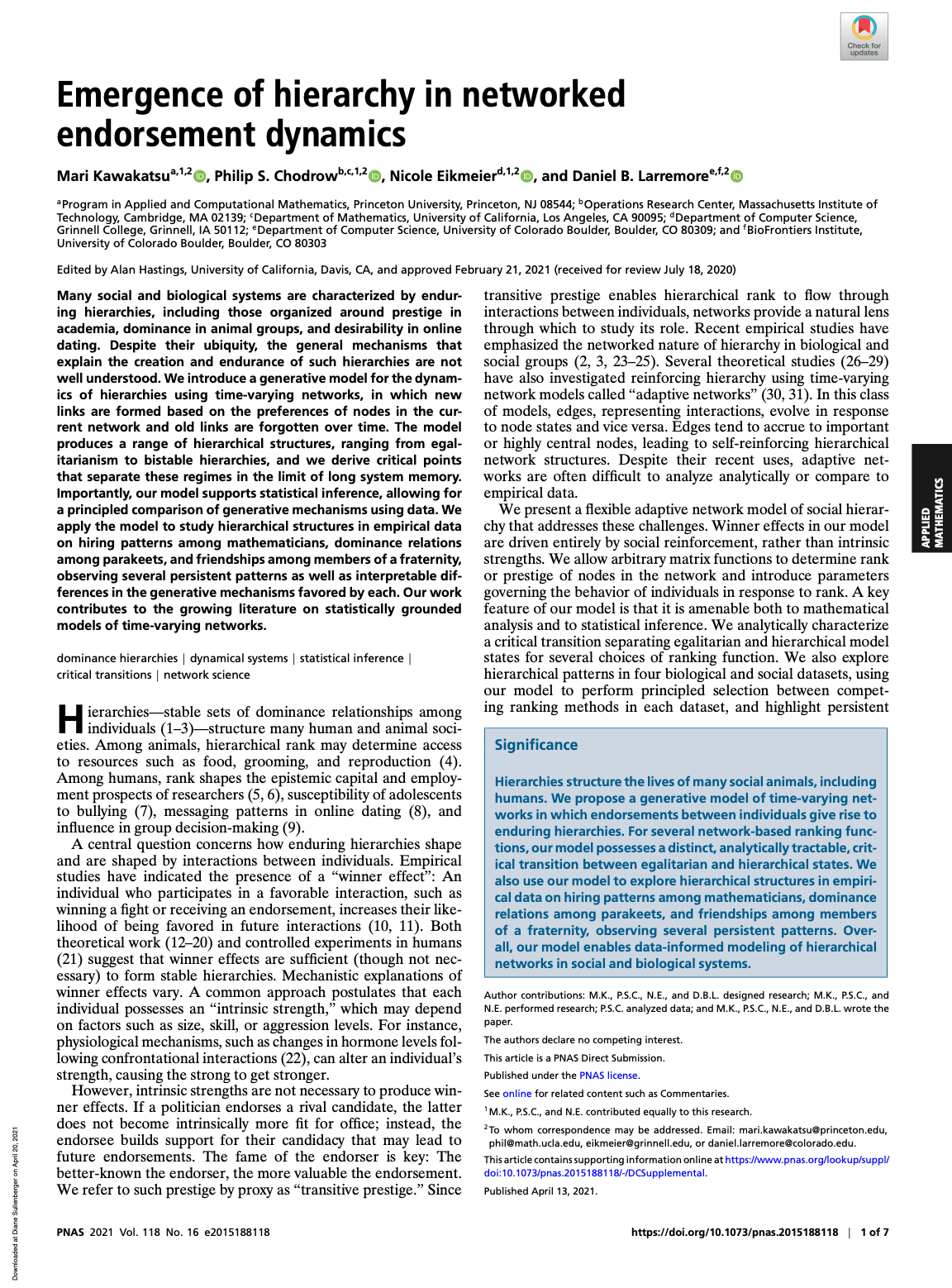### Two Ways to Start Projects

##### Idea First

“Here’s a cool idea!
What/who do I need to do in order to achieve this?”

##### People First

“Here are some people I like!
What cool things can we achieve together?”

I do both of these, but I have the most fun when I start with people.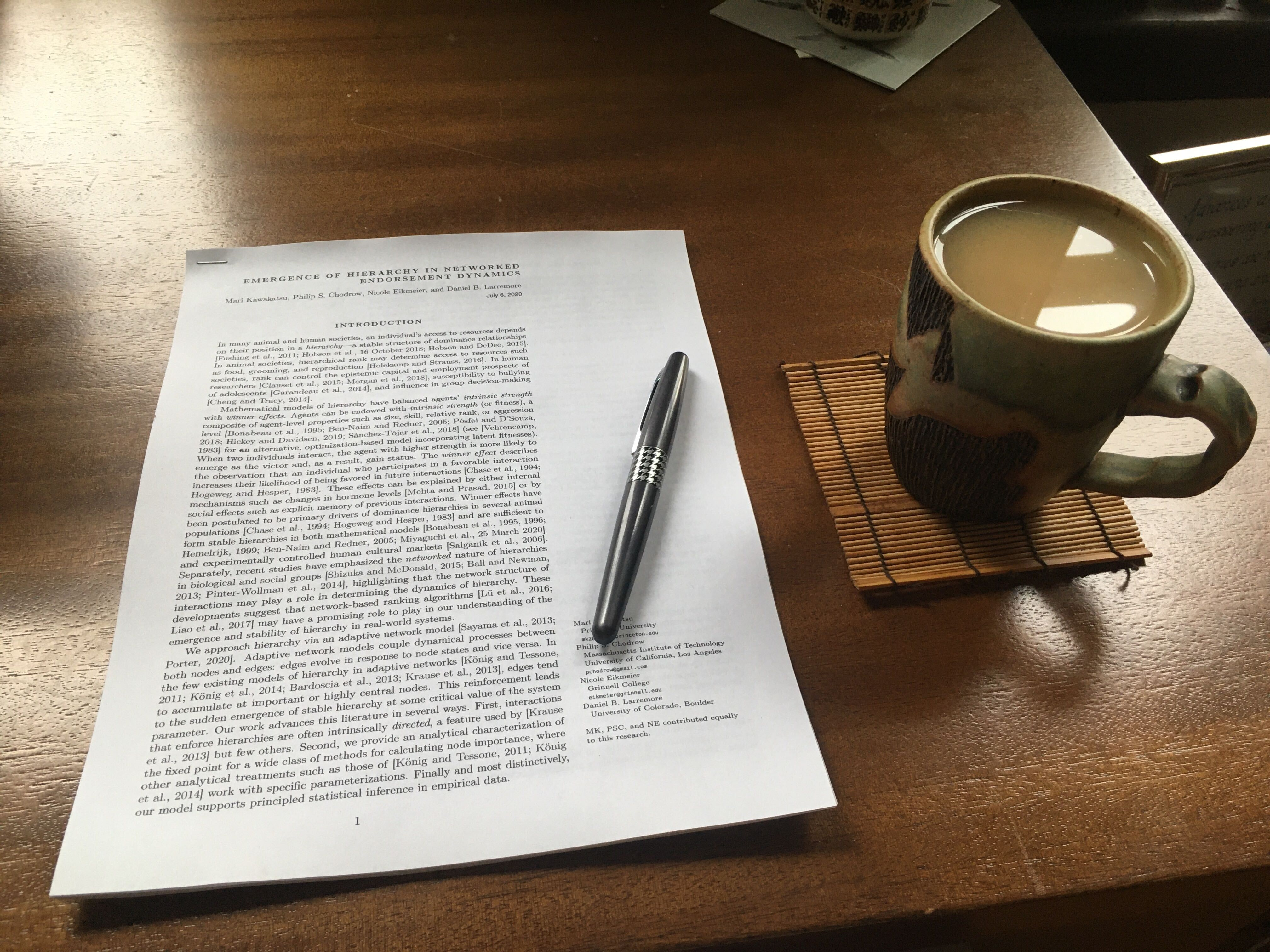##### Reflections on interdisciplinary applied mathbeing a human in science

Find people worth your trust, and trust them.

Be thankful, and say so.

Check in often.

Take time to see things from multiple angles.

Laugh. A lot.### Thanks!

##### Awesome Collaborators

Mari Kawakatsu
UPenn and Princeton

Nicole Eikmeier
Grinnell

Dan Larremore
CU Boulder